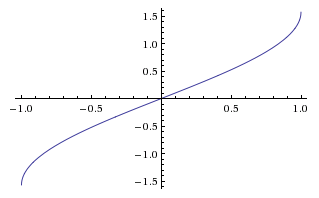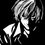# Astonishing Property of Inverse Trigonometric FunctionsI'm not sure if someone has noticed it before or not.

But anyways, I found this result today,

$\Re{(\mbox{arcsin } x)} = \left\{ \begin{array}{rl} \frac{\pi}{2} & \forall\ x \geq 1 \\ -\frac{\pi}{2} & \forall\ x \leq -1 \end{array} \right.$

$\Re{(\mbox{arccos } x)} = \left\{ \begin{array}{rl} 0 & \forall\ x \geq 1 \\ \pi & \forall\ x \leq -1 \end{array} \right.$

As @megh choksi suggested in my previous note, I'll not be sharing the proof right now. Instead, I'll wait for a few days for others to try.

Hint - You may read this wiki - Generalizing The Circular FunctionsNote by Kishlaya Jaiswal
6 years, 1 month ago

This discussion board is a place to discuss our Daily Challenges and the math and science related to those challenges. Explanations are more than just a solution — they should explain the steps and thinking strategies that you used to obtain the solution. Comments should further the discussion of math and science.

When posting on Brilliant:

• Use the emojis to react to an explanation, whether you're congratulating a job well done , or just really confused .
• Ask specific questions about the challenge or the steps in somebody's explanation. Well-posed questions can add a lot to the discussion, but posting "I don't understand!" doesn't help anyone.
• Try to contribute something new to the discussion, whether it is an extension, generalization or other idea related to the challenge.
• Stay on topic — we're all here to learn more about math and science, not to hear about your favorite get-rich-quick scheme or current world events.

MarkdownAppears as
*italics* or _italics_ italics
**bold** or __bold__ bold
- bulleted- list
• bulleted
• list
1. numbered2. list
1. numbered
2. list
Note: you must add a full line of space before and after lists for them to show up correctly
paragraph 1paragraph 2

paragraph 1

paragraph 2

[example link](https://brilliant.org)example link
> This is a quote
This is a quote
    # I indented these lines
# 4 spaces, and now they show
# up as a code block.

print "hello world"
# I indented these lines
# 4 spaces, and now they show
# up as a code block.

print "hello world"
MathAppears as
Remember to wrap math in $$ ... $$ or $ ... $ to ensure proper formatting.
2 \times 3 $2 \times 3$
2^{34} $2^{34}$
a_{i-1} $a_{i-1}$
\frac{2}{3} $\frac{2}{3}$
\sqrt{2} $\sqrt{2}$
\sum_{i=1}^3 $\sum_{i=1}^3$
\sin \theta $\sin \theta$
\boxed{123} $\boxed{123}$

## Comments

Sort by:

Top Newest

I'll just prove for $\Re(\arcsin(x)) = \frac{\pi}{2} \forall x \geq 1$ as a HINT for others to prove the rest $\ddot\smile$

We all know that $\arcsin (n) = -i\cdot ln(i\cdot n \pm \sqrt{1-n^{2}}) \\= -i\cdot ln(i\cdot n \pm i\sqrt{m} \\= -i\cdot ln(i(n \pm \sqrt{m}))$

Now we use $e^{ix} = cos(x) + i\cdot sin(x) \\ \quad \text{and} \quad \\= ln(i\cdot x) = ln(x\cdot e^{i\cdot \frac{\pi}{2}}) \\= -i\cdot ( ln(x) + i\cdot \frac{\pi}{2}) \\= \frac{\pi}{2} - ln(x)$

So we get $\\ \rightarrow \frac{\pi}{2} - i\cdot ln(n \pm \sqrt{m})$

Therefore $\Re(\arcsin(x)) = \frac{\pi}{2}$

- 6 years, 1 month ago

Log in to reply

Perfect!!! And similarly, we can work out the other relations too.

- 6 years, 1 month ago

Log in to reply

@Jake Lai Is it possible to make the fonts look big ?

- 6 years, 1 month ago

Log in to reply

Can I say this ?

$\Re{(\mbox{arcsin } x)} = \Re(\dfrac{\pi}{2} - cos^{-1} x)$

Thus $\dfrac{\pi}{2}$

- 6 years, 1 month ago

Log in to reply

Yep, but not exactly because there will be a contradiction.

Because $\Re\left(\frac{\pi}{2} - \cos^{-1}x \right) = \frac{\pi}{2} - \Re\left(\cos^{-1}x \right)$

And then you need to make assumption that $\Re\left(\cos^{-1}x \right) = 0$

And then to prove the above assumption, you will again need $\Re(\cos^{-1}x) = \Re\left(\frac{\pi}{2} - \sin^{-1}x \right)$

- 6 years, 1 month ago

Log in to reply

I mean for x>0 , arc(cosx) will always be imaginary , so real part of will be pi/2.

For $cos^{-1} x$ , the same way you proved,

$cos^{-1} x = ilog(x + i\sqrt{1 - x^2})$

For imaginary as well as real , it gives

$cos^{-1} x + sin^{-1} x = \dfrac{\pi}{2}$

Please correct me if I am wrong

- 6 years, 1 month ago

Log in to reply

@U Z Hmm, that seems perfectly reasonable.

- 6 years, 1 month ago

Log in to reply

Sorry for the suggestion , now as you wish please you post a wiki on it , will enjoy learning from you.

A request can you post a wiki on double summation.

- 6 years, 1 month ago

Log in to reply

@U Z Actually, I'll add that to the existing wiki itself.

And yes, I'll try my best to post a wiki on double summation as soon as I get time because I've my board examinations going on so it's a little difficult. Ok, so I'll write little by little each day and once the article gets completed, I'll be posting it.

- 6 years, 1 month ago

Log in to reply

- 6 years, 1 month ago

Log in to reply

Yeah , I'm on it . And check out my latest problem Kishlaya , it might be of significant interest to you !!!

- 6 years, 1 month ago

Log in to reply

Wow, that's an amazing problem and even the title being more amazing. And now, you're completely flattering me. Honestly speaking, I don't deserve that much brag.

You all (each and every Brilliantian) has been my inspiration. $:)$

- 6 years, 1 month ago

Log in to reply

Hey, I have a series of questions planned in which I'll be using concepts I have learned from you . So where do you want me to provide the links to those questions ? Seeing currently you won't get them as soon as I post them .

- 6 years, 1 month ago

Log in to reply

Hi Kishlaya , you considering the aspect of doing Research in Maths ? You'd do well . Given the standard of your questions, the ideas and solving techniques that you come up with I think I know who's gonna come out to be the AIR 1 in JEE Advance in a few months . Don't you think so too ?

- 6 years, 1 month ago

Log in to reply

Even I think, I know who's gonna be JEE AIR 1.

.

.

.

.

.

Ofcourse, it's you and who else $:)$

- 6 years, 1 month ago

Log in to reply

Dude there is a lot of competition and I can cite examples from Brilliant also with you , Pranjal , Ronak, Megh , Deepanshu topping the list ? Plus Physics and Inorganic Chemistry are Barriers that I have to overcome .

- 6 years, 1 month ago

Log in to reply

Same is the story with me. I find Chemistry to be the biggest hurdle that I have to cross anyhow, to get a good rank in JEE.

- 6 years, 1 month ago

Log in to reply

Well, I wish you the Best of Luck and hope that we both get into the same IIT and a good one at that !!

- 6 years, 1 month ago

Log in to reply

Thank you .

- 6 years, 1 month ago

Log in to reply

×

Problem Loading...

Note Loading...

Set Loading...